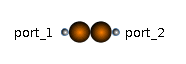Heated Pipe - MapleSim Help

Heated Pipe

Pipe with heat exchangeDescription The Heated Pipe component is a two-port thermal-fluid component that models a pipe with friction loss, head loss, and heat exchange with a heat port. The Medium parameter specifies the name of a Thermal-Fluid Media record, which must be in the model at the same or higer level. The Use default medium boolean parameter, when true, sets the name of the Medium parameter to fluidMedium1, which corresponds to the default name of a fluid medium record. To use a different name, uncheck the box and enter the name in the parameter input that appears.Equations ${p}_{\mathrm{drop}}=\Delta {p}_{\mathrm{lam}}\frac{{V}_{\mathrm{flow}}}{{V}_{{\mathrm{flow}}_{\mathrm{lam}}}}+\left\{\begin{array}{cc}+k{\left({V}_{\mathrm{flow}}-{V}_{{\mathrm{flow}}_{\mathrm{lam}}}\right)}^{2}& {V}_{{\mathrm{flow}}_{\mathrm{lam}}}<{V}_{\mathrm{flow}}\\ -k{\left({V}_{\mathrm{flow}}+{V}_{{\mathrm{flow}}_{\mathrm{lam}}}\right)}^{2}& {V}_{\mathrm{flow}}<-{V}_{{\mathrm{flow}}_{\mathrm{lam}}}\\ 0& \mathrm{otherwise}\end{array}$ $h={c}_{p}T$ $\Delta p={h}_{g}\mathrm{\rho }{g}_{n}+{p}_{\mathrm{drop}}$ ${Q}_{\mathrm{flow}}={Q}_{{\mathrm{flow}}_{\mathrm{port}}}+{Q}_{\mathrm{friction}}$ ${Q}_{\mathrm{friction}}={f}_{L}{V}_{\mathrm{flow}}{p}_{\mathrm{drop}}$ ${T}_{\mathrm{heatPort}}={T}_{q}$Variables

 Name Units Description Modelica ID $\Delta p$ $\mathrm{Pa}$ Pressure drop a->b dp ${p}_{\mathrm{drop}}$ $\mathrm{Pa}$ pressureDrop ${Q}_{\mathrm{flow}}$ $W$ Heat exchange with ambient Q_flow ${Q}_{\mathrm{friction}}$ $W$ Q_friction $T$ $K$ Outlet temperature of medium T $\Delta T$ $K$ Temperature increase of coolant in flow direction dT ${T}_{a}$ $K$ Temperature at flowPort_a T_a ${T}_{b}$ $K$ Temperature at flowPort_b T_b ${V}_{\mathrm{flow}}$ $\frac{{m}^{3}}{s}$ Volume flow from a to b V_flowConnections

 Name Description Modelica ID ${\mathrm{flowPort}}_{a}$ flowPort_a ${\mathrm{flowPort}}_{b}$ flowPort_b $\mathrm{heatPort}$ heatPortParameters

 Name Default Units Description Modelica ID $\mathrm{fix}{T}_{0}$ $\mathrm{false}$ True (checked) means ${T}_{0}$ is enforced, otherwise it is a guess T0fixed ${f}_{L}$ $0$ $1$ Part of friction losses fed to medium frictionLoss ${h}_{g}$ $0$ $m$ Geodetic height (height difference from flowPort_a to flowPort_b) h_g $m$ $1$ $\mathrm{kg}$ Mass of medium m $\mathrm{medium}$ fluidMedium medium $\Delta {p}_{\mathrm{lam}}$ $0.1$ $\mathrm{Pa}$ Laminar pressure drop dpLaminar $\Delta {p}_{\mathrm{nom}}$ $1$ $\mathrm{Pa}$ Nominal pressure drop dpNominal ${T}_{0}$ $293.15$ $K$ Initial temperature of medium T0 $\mathrm{tapT}$ $1$ $1$ Defines temperature of heat port; 0 = inlet temperature, 1 = outlet temperature tapT ${V}_{{\mathrm{flow}}_{\mathrm{lam}}}$ $0.1$ $\frac{{m}^{3}}{s}$ Laminar volume flow V_flowLaminar ${V}_{{\mathrm{flow}}_{\mathrm{nom}}}$ $1$ $\frac{{m}^{3}}{s}$ Nominal volume flow V_flowNominalModelica Standard Library The component described in this topic is from the Modelica Standard Library. To view the original documentation, which includes author and copyright information, click here.